# Intrusion Detection System Using Machine Learning Algorithms

Problem Statement: The task is to build a network intrusion detector, a predictive model capable of distinguishing between bad connections, called intrusions or attacks, and good normal connections.

Introduction:
Intrusion Detection System is a software application to detect network intrusion using various machine learning algorithms.IDS monitors a network or system for malicious activity and protects a computer network from unauthorized access from users, including perhaps insider. The intrusion detector learning task is to build a predictive model (i.e. a classifier) capable of distinguishing between ‘bad connections’ (intrusion/attacks) and a ‘good (normal) connections’.

Attacks fall into four main categories:

• #DOS: denial-of-service, e.g. syn flood;
• #R2L: unauthorized access from a remote machine, e.g. guessing password;
• #U2R: unauthorized access to local superuser (root) privileges, e.g., various “buffer overflow” attacks;
• #probing: surveillance and another probing, e.g., port scanning.

Dataset Used : KDD Cup 1999 dataset

Dataset Description: Data files:

• kddcup.names : A list of features.
• kddcup.data.gz : The full data set
• kddcup.data_10_percent.gz : A 10% subset.
• kddcup.newtestdata_10_percent_unlabeled.gz
• kddcup.testdata.unlabeled.gz
• kddcup.testdata.unlabeled_10_percent.gz
• corrected.gz : Test data with corrected labels.
• training_attack_types : A list of intrusion types.
• typo-correction.txt : A brief note on a typo in the data set that has been corrected

Features:

Table 1: Basic features of individual TCP connections.

Table 2: Content features within a connection suggested by domain knowledge.

Table 3: Traffic features computed using a two-second time window.

Various Algorithms Applied: Guassian Naive Bayes, Decision Tree, Random Fprest, Support Vector Machine, Logistic Regression.

Approach Used: I have applied various classification algorithms that are mentioned above on the KDD dataset and compare there results to build a predictive model.

Step 1 – Data Preprocessing:

Code: Importing libraries and reading features list from ‘kddcup.names’ file.

 `import` `os ` `import` `pandas as pd ` `import` `numpy as np ` `import` `matplotlib.pyplot as plt ` `import` `seaborn as sns ` `import` `time ` ` `  `# reading features list ` `with ``open``(``"..\\kddcup.names"``, ``'r'``) as f: ` `    ``print``(f.read()) `

Code: Appending columns to the dataset and adding a new column name ‘target’ to the dataset.

 `  ` `cols ``=``"""duration, ` `protocol_type, ` `service, ` `flag, ` `src_bytes, ` `dst_bytes, ` `land, ` `wrong_fragment, ` `urgent, ` `hot, ` `num_failed_logins, ` `logged_in, ` `num_compromised, ` `root_shell, ` `su_attempted, ` `num_root, ` `num_file_creations, ` `num_shells, ` `num_access_files, ` `num_outbound_cmds, ` `is_host_login, ` `is_guest_login, ` `count, ` `srv_count, ` `serror_rate, ` `srv_serror_rate, ` `rerror_rate, ` `srv_rerror_rate, ` `same_srv_rate, ` `diff_srv_rate, ` `srv_diff_host_rate, ` `dst_host_count, ` `dst_host_srv_count, ` `dst_host_same_srv_rate, ` `dst_host_diff_srv_rate, ` `dst_host_same_src_port_rate, ` `dst_host_srv_diff_host_rate, ` `dst_host_serror_rate, ` `dst_host_srv_serror_rate, ` `dst_host_rerror_rate, ` `dst_host_srv_rerror_rate"""` ` `  `columns ``=``[] ` `for` `c ``in` `cols.split(``', '``): ` `    ``if``(c.strip()): ` `       ``columns.append(c.strip()) ` ` `  `columns.append(``'target'``) ` `print``(``len``(columns)) `

Output:

`42`

 `  ` `with ``open``(``"..\\training_attack_types"``, ``'r'``) as f: ` `    ``print``(f.read()) `

Output:

```back dos
buffer_overflow u2r
ftp_write r2l
guess_passwd r2l
imap r2l
ipsweep probe
land dos
multihop r2l
neptune dos
nmap probe
perl u2r
phf r2l
pod dos
portsweep probe
rootkit u2r
satan probe
smurf dos
spy r2l
teardrop dos
warezclient r2l
warezmaster r2l```

Code: Creating a dictionary of attack_types

 `   ` `attacks_types ``=` `{ ` `    ``'normal'``: ``'normal'``, ` `'back'``: ``'dos'``, ` `'buffer_overflow'``: ``'u2r'``, ` `'ftp_write'``: ``'r2l'``, ` `'guess_passwd'``: ``'r2l'``, ` `'imap'``: ``'r2l'``, ` `'ipsweep'``: ``'probe'``, ` `'land'``: ``'dos'``, ` `'loadmodule'``: ``'u2r'``, ` `'multihop'``: ``'r2l'``, ` `'neptune'``: ``'dos'``, ` `'nmap'``: ``'probe'``, ` `'perl'``: ``'u2r'``, ` `'phf'``: ``'r2l'``, ` `'pod'``: ``'dos'``, ` `'portsweep'``: ``'probe'``, ` `'rootkit'``: ``'u2r'``, ` `'satan'``: ``'probe'``, ` `'smurf'``: ``'dos'``, ` `'spy'``: ``'r2l'``, ` `'teardrop'``: ``'dos'``, ` `'warezclient'``: ``'r2l'``, ` `'warezmaster'``: ``'r2l'``, ` `} `

Code: Reading the dataset(‘kddcup.data_10_percent.gz’) and adding Attack Type feature in the training dataset where attack type feature has 5 distinct values i.e. dos, normal, probe, r2l, u2r.

 `path ``=` `"..\\kddcup.data_10_percent.gz"` `df ``=` `pd.read_csv(path, names ``=` `columns) ` ` `  `# Adding Attack Type column ` `df[``'Attack Type'``] ``=` `df.target.``apply``(``lambda` `r:attacks_types[r[:``-``1``]]) ` `df.head() ` ` `

Code: Shape of dataframe and getting data type of each feature

 `  ` `df.shape `

Output:

` (494021, 43)`

Code: Finding missing values of all features.

 `df.isnull().``sum``()`

Output:

```duration                       0
protocol_type                  0
service                        0
flag                           0
src_bytes                      0
dst_bytes                      0
land                           0
wrong_fragment                 0
urgent                         0
hot                            0
logged_in                      0
num_compromised                0
root_shell                     0
su_attempted                   0
num_root                       0
num_file_creations             0
num_shells                     0
num_access_files               0
num_outbound_cmds              0
count                          0
srv_count                      0
serror_rate                    0
srv_serror_rate                0
rerror_rate                    0
srv_rerror_rate                0
same_srv_rate                  0
diff_srv_rate                  0
srv_diff_host_rate             0
dst_host_count                 0
dst_host_srv_count             0
dst_host_same_srv_rate         0
dst_host_diff_srv_rate         0
dst_host_same_src_port_rate    0
dst_host_srv_diff_host_rate    0
dst_host_serror_rate           0
dst_host_srv_serror_rate       0
dst_host_rerror_rate           0
dst_host_srv_rerror_rate       0
target                         0
Attack Type                    0
dtype: int64```

No missing value found, so we can further proceed to our next step.

Code: Finding Categorical Features

 `# Finding categorical features ` `num_cols ``=` `df._get_numeric_data().columns ` ` `  `cate_cols ``=` `list``(``set``(df.columns)``-``set``(num_cols)) ` `cate_cols.remove(``'target'``) ` `cate_cols.remove(``'Attack Type'``) ` ` `  `cate_cols ` ` `

Output:

` ['service', 'flag', 'protocol_type']`

Visualizing Categorical Features using bar graphProtocol type: We notice that ICMP is the most present in the used data, then TCP and almost 20000 packets of UDP typelogged_in (1 if successfully logged in; 0 otherwise): We notice that just 70000 packets are successfully logged in.

Target Feature Distribution: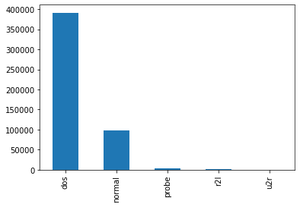Attack Type(The attack types grouped by attack, it’s what we will predict)

Code: Data Correlation – Find the highly correlated variables using heatmap and ignore them for analysis.

 `  ` ` `  `df ``=` `df.dropna(``'columns'``)``# drop columns with NaN ` ` `  `df ``=` `df[[col ``for` `col ``in` `df ``if` `df[col].nunique() > ``1``]]``# keep columns where there are more than 1 unique values ` ` `  `corr ``=` `df.corr() ` ` `  `plt.figure(figsize ``=``(``15``, ``12``)) ` ` `  `sns.heatmap(corr) ` ` `  `plt.show() ` ` `

Output: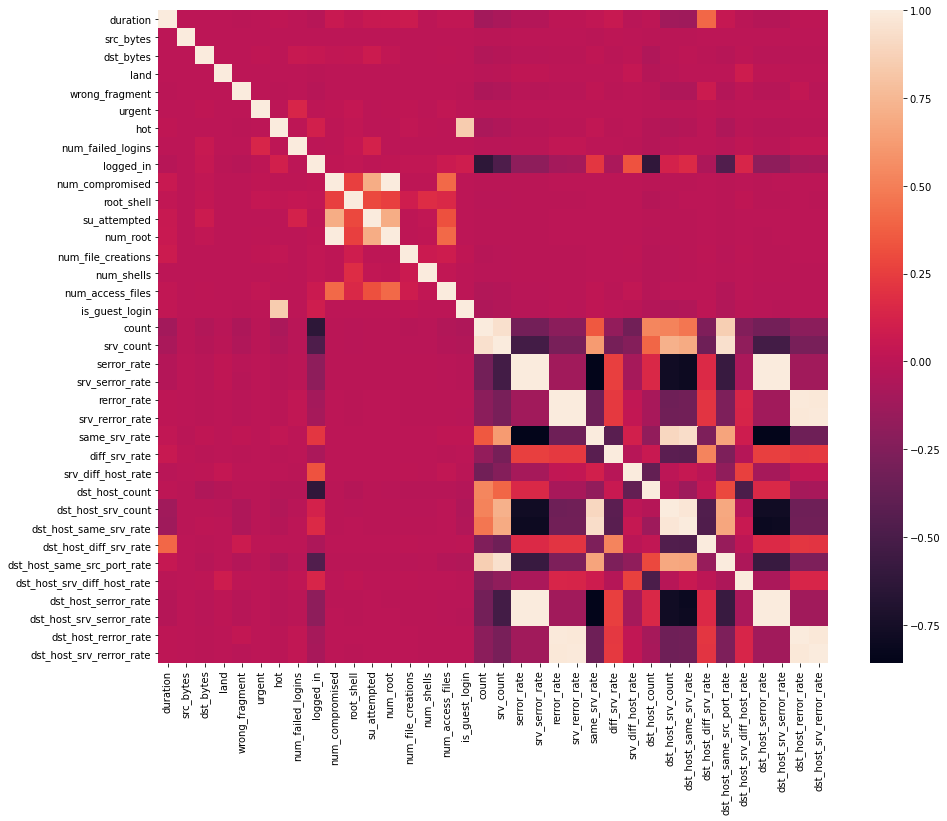Code:

 `  ` ` `  `# This variable is highly correlated with num_compromised and should be ignored for analysis. ` `#(Correlation = 0.9938277978738366) ` `df.drop(``'num_root'``, axis ``=` `1``, inplace ``=` `True``) ` ` `  `# This variable is highly correlated with serror_rate and should be ignored for analysis. ` `#(Correlation = 0.9983615072725952) ` `df.drop(``'srv_serror_rate'``, axis ``=` `1``, inplace ``=` `True``) ` ` `  `# This variable is highly correlated with rerror_rate and should be ignored for analysis. ` `#(Correlation = 0.9947309539817937) ` `df.drop(``'srv_rerror_rate'``, axis ``=` `1``, inplace ``=` `True``) ` ` `  `# This variable is highly correlated with srv_serror_rate and should be ignored for analysis. ` `#(Correlation = 0.9993041091850098) ` `df.drop(``'dst_host_srv_serror_rate'``, axis ``=` `1``, inplace ``=` `True``) ` ` `  `# This variable is highly correlated with rerror_rate and should be ignored for analysis. ` `#(Correlation = 0.9869947924956001) ` `df.drop(``'dst_host_serror_rate'``, axis ``=` `1``, inplace ``=` `True``) ` ` `  `# This variable is highly correlated with srv_rerror_rate and should be ignored for analysis. ` `#(Correlation = 0.9821663427308375) ` `df.drop(``'dst_host_rerror_rate'``, axis ``=` `1``, inplace ``=` `True``) ` ` `  `# This variable is highly correlated with rerror_rate and should be ignored for analysis. ` `#(Correlation = 0.9851995540751249) ` `df.drop(``'dst_host_srv_rerror_rate'``, axis ``=` `1``, inplace ``=` `True``) ` ` `  `# This variable is highly correlated with srv_rerror_rate and should be ignored for analysis. ` `#(Correlation = 0.9865705438845669) ` `df.drop(``'dst_host_same_srv_rate'``, axis ``=` `1``, inplace ``=` `True``) ` ` `

Output:

Code: Feature Mapping – Apply feature mapping on features such as : ‘protocol_type’ & ‘flag’.

 `  ` `# protocol_type feature mapping ` `pmap ``=` `{``'icmp'``:``0``, ``'tcp'``:``1``, ``'udp'``:``2``} ` `df[``'protocol_type'``] ``=` `df[``'protocol_type'``].``map``(pmap) `

Code:

 `  ` `# flag feature mapping ` `fmap ``=` `{``'SF'``:``0``, ``'S0'``:``1``, ``'REJ'``:``2``, ``'RSTR'``:``3``, ``'RSTO'``:``4``, ``'SH'``:``5``, ``'S1'``:``6``, ``'S2'``:``7``, ``'RSTOS0'``:``8``, ``'S3'``:``9``, ``'OTH'``:``10``} ` `df[``'flag'``] ``=` `df[``'flag'``].``map``(fmap) ` ` `

Output:

Code: Remove irrelevant features such as ‘service’ before modelling

 `  ` `df.drop(``'service'``, axis ``=` `1``, inplace ``=` `True``) `

Step 2 – Modelling

Code: Importing libraries and splitting the dataset

 `  ` `from` `sklearn.model_selection ``import` `train_test_split ` `from` `sklearn.preprocessing ``import` `MinMaxScaler ` ` `

Code:

 `  ` `# Splitting the dataset ` `df ``=` `df.drop([``'target'``, ], axis ``=` `1``) ` `print``(df.shape) ` ` `  `# Target variable and train set ` `y ``=` `df[[``'Attack Type'``]] ` `X ``=` `df.drop([``'Attack Type'``, ], axis ``=` `1``) ` ` `  `sc ``=` `MinMaxScaler() ` `X ``=` `sc.fit_transform(X) ` ` `  `# Split test and train data  ` `X_train, X_test, y_train, y_test ``=` `train_test_split(X, y, test_size ``=` `0.33``, random_state ``=` `42``) ` `print``(X_train.shape, X_test.shape) ` `print``(y_train.shape, y_test.shape) ` ` `

Output:

```(494021, 31)
(330994, 30) (163027, 30)
(330994, 1) (163027, 1)```

Apply various machine learning classification algorithms such as Support Vector Machines, Random Forest, Naive Bayes, Decision Tree, Logistic Regression to create different models.

Code: Python implementation of Guassian Naive Bayes

 `  ` `# Gaussian Naive Bayes ` `from` `sklearn.naive_bayes ``import` `GaussianNB ` `from` `sklearn.metrics ``import` `accuracy_score ` ` `  `clfg ``=` `GaussianNB() ` `start_time ``=` `time.time() ` `clfg.fit(X_train, y_train.values.ravel()) ` `end_time ``=` `time.time() ` `print``(``"Training time: "``, end_time``-``start_time) ` ` `

Output:

`Training time:  1.1145250797271729`

Code:

 `  ` `start_time ``=` `time.time() ` `y_test_pred ``=` `clfg.predict(X_train) ` `end_time ``=` `time.time() ` `print``(``"Testing time: "``, end_time``-``start_time) ` ` `

Output:

`Testing time:  1.543299674987793`

Code:

 `  ` `print``(``"Train score is:"``, clfg.score(X_train, y_train)) ` `print``(``"Test score is:"``, clfg.score(X_test, y_test)) ` ` `

Output:

```Train score is: 0.8795114110829804
Test score is: 0.8790384414851528```

Code: Python implementation of Decision Tree

 `  ` `# Decision Tree  ` `from` `sklearn.tree ``import` `DecisionTreeClassifier ` ` `  `clfd ``=` `DecisionTreeClassifier(criterion ``=``"entropy"``, max_depth ``=` `4``) ` `start_time ``=` `time.time() ` `clfd.fit(X_train, y_train.values.ravel()) ` `end_time ``=` `time.time() ` `print``(``"Training time: "``, end_time``-``start_time) ` ` `

Output:

` Training time:  2.4408750534057617`

 `  ` `start_time ``=` `time.time() ` `y_test_pred ``=` `clfd.predict(X_train) ` `end_time ``=` `time.time() ` `print``(``"Testing time: "``, end_time``-``start_time) ` ` `

Output:

`Testing time:  0.1487727165222168`

 `  ` `print``(``"Train score is:"``, clfd.score(X_train, y_train)) ` `print``(``"Test score is:"``, clfd.score(X_test, y_test)) ` ` `

Output:

```Train score is: 0.9905829108684749
Test score is: 0.9905230421954646```

Code: Python code implementation of Random Forest

 `  ` `from` `sklearn.ensemble ``import` `RandomForestClassifier ` ` `  `clfr ``=` `RandomForestClassifier(n_estimators ``=` `30``) ` `start_time ``=` `time.time() ` `clfr.fit(X_train, y_train.values.ravel()) ` `end_time ``=` `time.time() ` `print``(``"Training time: "``, end_time``-``start_time) ` ` `

Output:

` Training time:  17.084914684295654`

 `  ` `start_time ``=` `time.time() ` `y_test_pred ``=` `clfr.predict(X_train) ` `end_time ``=` `time.time() ` `print``(``"Testing time: "``, end_time``-``start_time) ` ` `

Output:

`Testing time:  0.1487727165222168`

 `  ` `print``(``"Train score is:"``, clfr.score(X_train, y_train)) ` `print``(``"Test score is:"``, clfr.score(X_test, y_test)) ` ` `

Output:

```Train score is: 0.99997583037759
Test score is: 0.9996933023364228```

Code: Python implementation of Support Vector Classifier

 `  ` `from` `sklearn.svm ``import` `SVC ` ` `  `clfs ``=` `SVC(gamma ``=` `'scale'``) ` `start_time ``=` `time.time() ` `clfs.fit(X_train, y_train.values.ravel()) ` `end_time ``=` `time.time() ` `print``(``"Training time: "``, end_time``-``start_time) ` ` `

Output:

`Training time:  218.26840996742249`

Code:

 `  ` `start_time ``=` `time.time() ` `y_test_pred ``=` `clfs.predict(X_train) ` `end_time ``=` `time.time() ` `print``(``"Testing time: "``, end_time``-``start_time) ` ` `

Output:

`Testing time:  126.5087513923645`

Code:

 `  ` `print``(``"Train score is:"``, clfs.score(X_train, y_train)) ` `print``(``"Test score is:"``, clfs.score(X_test, y_test)) ` ` `

Output:

```Train score is: 0.9987552644458811
Test score is: 0.9987916112055059```

Code: Python implementation of Logistic Regression

 `  ` `from` `sklearn.linear_model ``import` `LogisticRegression ` ` `  `clfl ``=` `LogisticRegression(max_iter ``=` `1200000``) ` `start_time ``=` `time.time() ` `clfl.fit(X_train, y_train.values.ravel()) ` `end_time ``=` `time.time() ` `print``(``"Training time: "``, end_time``-``start_time) ` ` `

Output:

`Training time:  92.94222283363342`

Code:

 `  ` `start_time ``=` `time.time() ` `y_test_pred ``=` `clfl.predict(X_train) ` `end_time ``=` `time.time() ` `print``(``"Testing time: "``, end_time``-``start_time) ` ` `

Output:

`Testing time:  0.09605908393859863`

Code:

 `  ` `print``(``"Train score is:"``, clfl.score(X_train, y_train)) ` `print``(``"Test score is:"``, clfl.score(X_test, y_test)) ` ` `

Output:

```Train score is: 0.9935285835997028
Test score is: 0.9935286792985211```

Code: Python implementation of Gradient Descent

 `  ` `from` `sklearn.ensemble ``import` `GradientBoostingClassifier ` ` `  `clfg ``=` `GradientBoostingClassifier(random_state ``=` `0``) ` `start_time ``=` `time.time() ` `clfg.fit(X_train, y_train.values.ravel()) ` `end_time ``=` `time.time() ` `print``(``"Training time: "``, end_time``-``start_time) ` ` `

Output:

`Training time:  633.2290260791779`

 `  ` `start_time ``=` `time.time() ` `y_test_pred ``=` `clfg.predict(X_train) ` `end_time ``=` `time.time() ` `print``(``"Testing time: "``, end_time``-``start_time) ` ` `

Output:

`Testing time:  2.9503915309906006`

 `  ` `print``(``"Train score is:"``, clfg.score(X_train, y_train)) ` `print``(``"Test score is:"``, clfg.score(X_test, y_test)) ` ` `

Output:

```Train score is: 0.9979304760811374
Test score is: 0.9977181693829856```

Code: Analyse the training and testing accuracy of each model.

 `  ` `names ``=` `[``'NB'``, ``'DT'``, ``'RF'``, ``'SVM'``, ``'LR'``, ``'GB'``] ` `values ``=` `[``87.951``, ``99.058``, ``99.997``, ``99.875``, ``99.352``, ``99.793``] ` `f ``=` `plt.figure(figsize ``=``(``15``, ``3``), num ``=` `10``) ` `plt.subplot(``131``) ` `plt.bar(names, values) `

Output: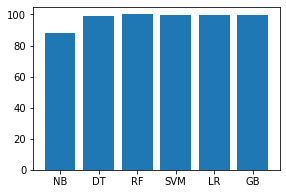Code:

 `  ` `names ``=` `[``'NB'``, ``'DT'``, ``'RF'``, ``'SVM'``, ``'LR'``, ``'GB'``] ` `values ``=` `[``87.903``, ``99.052``, ``99.969``, ``99.879``, ``99.352``, ``99.771``] ` `f ``=` `plt.figure(figsize ``=``(``15``, ``3``), num ``=` `10``) ` `plt.subplot(``131``) ` `plt.bar(names, values) ` ` `

Output: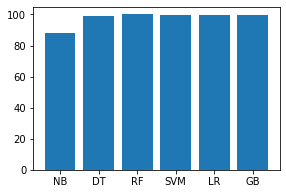Code: Analyse the training and testing time of each model.

 `  ` `names ``=` `[``'NB'``, ``'DT'``, ``'RF'``, ``'SVM'``, ``'LR'``, ``'GB'``] ` `values ``=` `[``1.11452``, ``2.44087``, ``17.08491``, ``218.26840``, ``92.94222``, ``633.229``] ` `f ``=` `plt.figure(figsize ``=``(``15``, ``3``), num ``=` `10``) ` `plt.subplot(``131``) ` `plt.bar(names, values) ` ` `

Output: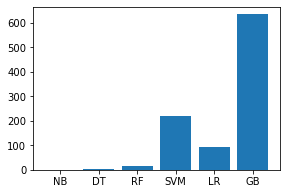Code:

 `  ` `names ``=` `[``'NB'``, ``'DT'``, ``'RF'``, ``'SVM'``, ``'LR'``, ``'GB'``] ` `values ``=` `[``1.54329``, ``0.14877``, ``0.199471``, ``126.50875``, ``0.09605``, ``2.95039``] ` `f ``=` `plt.figure(figsize ``=``(``15``, ``3``), num ``=` `10``) ` `plt.subplot(``131``) ` `plt.bar(names, values) ` ` `

Output: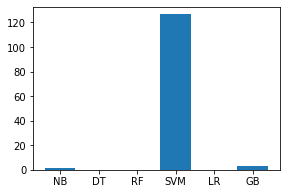Conclusion: The above analysis of different models states that the Decision Tree model best fits our data considering both accuracy and time complexity.

Links: The complete code is uploaded on my github account – https://github.com/mudgalabhay/intrusion-detection-system

Attention geek! Strengthen your foundations with the Python Programming Foundation Course and learn the basics.

To begin with, your interview preparations Enhance your Data Structures concepts with the Python DS Course.

My Personal Notes arrow_drop_upCheck out this Author's contributed articles.

If you like GeeksforGeeks and would like to contribute, you can also write an article using contribute.geeksforgeeks.org or mail your article to contribute@geeksforgeeks.org. See your article appearing on the GeeksforGeeks main page and help other Geeks.

Please Improve this article if you find anything incorrect by clicking on the "Improve Article" button below.

Article Tags :
Practice Tags :

Be the First to upvote.

Please write to us at contribute@geeksforgeeks.org to report any issue with the above content.